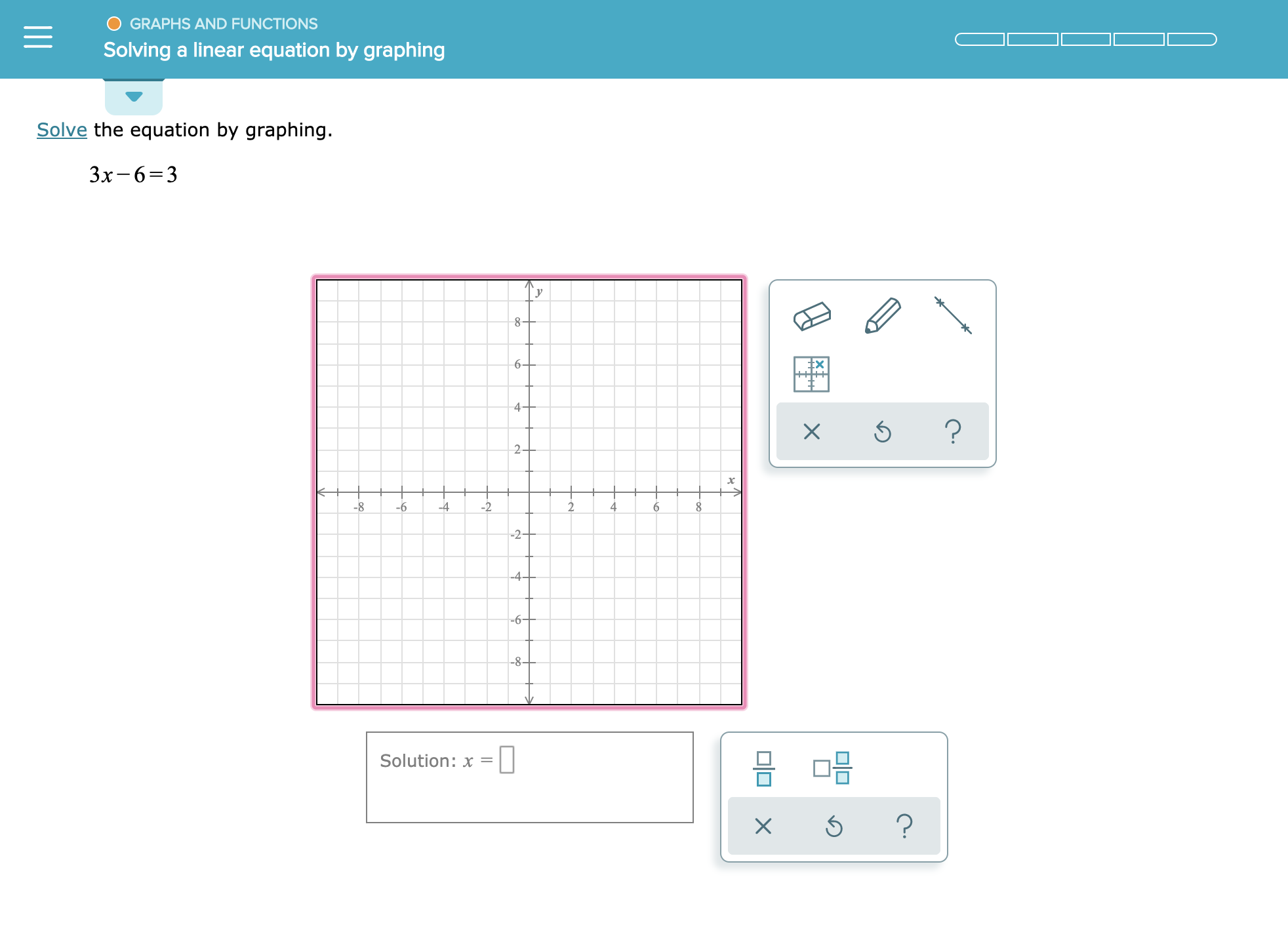# GRAPHS AND FUNCTIONS Solving a linear equation by graphing Solve the equation by graphing. 3x-6 3 6+ 4 ? X 2 -8 -4 -6 -2+ -4- -6- 믐 □믐 Solution: x = ? X

Question

See attachmenthelp_outlineImage TranscriptioncloseGRAPHS AND FUNCTIONS Solving a linear equation by graphing Solve the equation by graphing. 3x-6 3 6+ 4 ? X 2 -8 -4 -6 -2+ -4- -6- 믐 □믐 Solution: x = ? X fullscreen

### Want to see this answer and more?

Experts are waiting 24/7 to provide step-by-step solutions in as fast as 30 minutes!*

*Response times vary by subject and question complexity. Median response time is 34 minutes and may be longer for new subjects.
Tagged in
MathCalculus

### Other